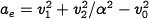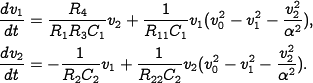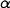Errata: In Exercise 4.12, some additional assumptions are required for oscillation

Location: page 129, line 6

As written, some additional assumptions of the values of the resistances and capacitances are needed in order to obtain an oscillation. For a simple fix, change the last line of the exercise to read

Show that, under suitable conditions on parameter values, the circuit gives an oscillation with a stable limit cycle with amplitude.

A better solution is to modify the exercise to read as follows:

An op amp circuit for an oscillator was shown in Exercise 3.5. The oscillatory solution for that linear circuit was stable but not asymptotically stable. A schematic of a modified circuit that has nonlinear elements is shown in the figure below (omitted; see text).

The modification is obtained by making a feedback around each operational amplifier that has capacitors using multipliers. The signalis the amplitude error. Show that the system is modeled byDetermineso that the the circuit gives an oscillation with a stable limit cycle with amplitude.

(Contributed by K. J. Astrom, 8 Mar 2011)